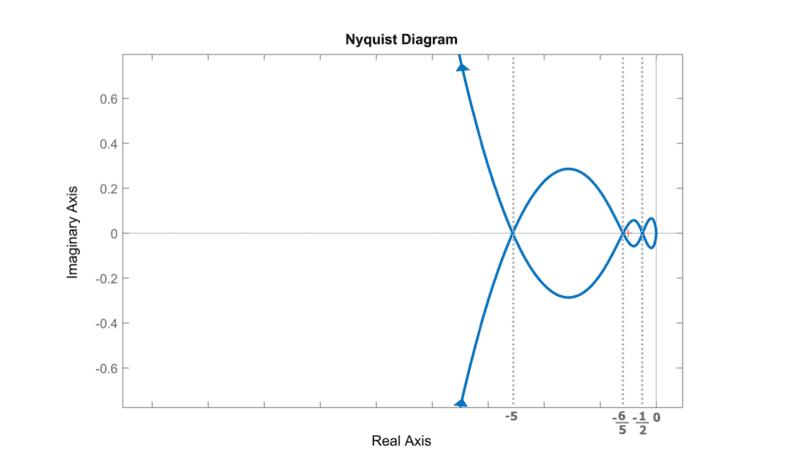# How to find the number of zeros in transfer function from the Nyquist diagram

• Engineering
Karl Karlsson
Homework Statement:
The Nyquist curve for a fifth order system with transfer function G(s) is given in the attached photo. Here we have plotted G (iω) for both positive and negative frequencies ω. The transfer function G(s) has no poles or zeros strictly in the right hemisphere
Relevant Equations:
None
The Nyquist curve for a fifth order system with transfer function G(s) is given in the attached photo. Here we have plotted G(iω) for both positive and negative frequencies ω. The transfer function G(s) has no poles or zeros strictly on the right side of the imaginary axisPoint −1 is marked with a (small) cross and can also be obtained via the scale on the Re-axis.

i) Use the Nyquist curve in Figure 3 to determine how many zeros the system has.
------------------------------------------------------------------------------------------------
My attempt:
Why the point -1 was given is for a later task when you are supposed to check stability which I might need help with later. Anyway, this is a problem on an old exam so I already know the answer is 2 but I don't know how they know that from the given nyquist diagram. I know what a nyquist diagram and bode plots are but I don't know how they got to that answer. We can see from the diagram that there is at least one zero since G(iw) goes through the origin. That zero is probably when w=0 because the diagram looks completely symmetrical around the real axis. But maybe the other zero is of the form a+wi, a is not 0. I would not be able to find a zeros that has a real part from the nyquist diagram. Of course we don't need to find the other zero they only ask us how many zeros there are but I don't know how to determine that there are 2 zeros.

Gold Member
They don't write in the solution of the exam why is it two?
I assume you are well acquainted with Nyquist curve criterion theorem here:
https://en.wikipedia.org/wiki/Nyquist_stability_criterion
It seems the theorem tells us that the number of clockwise encirclements of the curve over the origin must be the number of zeros of ##1+G(s)##, i.e a zero of this gives you: ##G(s*)=-1##. So if you find the number of encirclrments of the curve over -1 you'll get the number of zeros of ##G(s)##, seems reasonable doesn't it?

Karl Karlsson
They don't write in the solution of the exam why is it two?
They come to the conclusion that there are 2 zeros by ##wi## becoming dominant for large values of w and each ##wi## in the denominator gives a phase shift of -90° and each ##wi## in the numerator gives a phase shift of +90°. Thus there must be 2 zeros because you see that ##G(iw)## phase shift -270° for large w. The problem I have with it is that the expression will probably be approximately ##a\cdot(wi)^2/(b \cdot (wi) ^ 5 )##, ie we do not know if the constants a and b are negative and then we get a completely different phase shift. Then there are also many solutions, eg if G(s) were ##\frac{-s ^ 4} {(s + 1)(s + 2)(s + 3)(s + 4)(s + 5)}##, here you see that ##G (iw)## phase rotates -270 degrees for large values of w and there is only one zero (I do not know if they in the task count double root as two zeros)
So if you find the number of encirclrments of the curve over -1 you'll get the number of zeros of ##G(s)##, seems reasonable doesn't it?
The way I have learned it is that the number of poles with positive real part to the feedback system ##1/(1+G_0(s))## is equal to the number of poles with positive real part of ##G_0## plus the number of encirclements of the point -1 in a nyquist diagram. Not sure if that is in a nyquist diagram where w goes from ##-\inf## to ##\inf## or 0 to ##\inf##. Does anyone know which it is?

Gold Member
They may consider a zero with multiplicity 2 as two zeros.
I remember from my notes that in the proof of Nyquist criterion that zeros are counted with their multiplicities. The proof of the theorem goes through the argument theorem in complex analysis.